# Quickstart

If you're comfortable with Julia or have used another image-processing package before, this page may help you get started quickly. If some of the terms or concepts here seem strange, don't worry–-there are much more detailed explanations in the following sections.

## Images are just arrays

For most purposes, any AbstractArray can be treated as an image. For example, numeric array can be interpreted as a grayscale image.

julia> img = rand(4, 3)
4×3 Array{Float64,2}:
0.294863  0.603624   0.113032
0.696311  0.0443766  0.670174
0.332355  0.915681   0.140473
0.415085  0.372323   0.0813266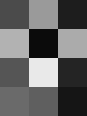We could also select a region-of-interest from a larger image

# generate an image that starts black in the upper left
# and gets bright in the lower right
img = Array(reshape(range(0,stop=1,length=10^4), 100, 100))
# make a copy
img_c = img[51:70, 21:70] # red
# make a view
img_v = @view img[16:35, 41:90] # blue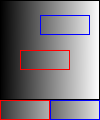As you might know, changing the value of a view would affect the original image, while changing that of a copy doesn't:

fill!(img_c, 1) # red region in original doesn't change
fill!(img_v, 0) # blue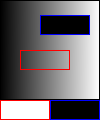Don't worry if you don't get the "image" result, that's expected and you'll learn how to automatically display an image later in JuliaImages.

using Unitful, AxisArrays
using Unitful: mm, s

img = AxisArray(rand(256, 256, 6, 50), (:x, :y, :z, :time), (0.4mm, 0.4mm, 1mm, 2s))

defines a 4d image (3 space dimensions plus one time dimension) with the specified name and physical pixel spacing for each coordinate. The AxisArrays package supports rich and efficient operations on such arrays, and can be useful to keep track of not just pixel spacing but the orientation convention used for multidimensional images.

JuliaImages interoperates smoothly with AxisArrays and many other packages. As further examples,

It is very easy to define new array types in Julia–and consequently specialized images or operations–and have them interoperate smoothly with the vast majority of functions in JuliaImages.

## Array elements are pixels (and vice versa)

Elements of image are called pixels; in JuliaImages we provide an abstraction on this concept. For example, we have Gray for grayscale image, RGB for RGB image, Lab for Lab image, and etc.

Creating a pixel is initializing a struct of that type:

Gray(0.0) # black
Gray(1.0) # white
RGB(1.0, 0.0, 0.0) # red
RGB(0.0, 1.0, 0.0) # green
RGB(0.0, 0.0, 1.0) # blue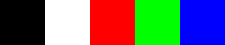and image is just an array of pixel objects:

julia> img_gray = rand(Gray, 2, 2)
2×2 Array{Gray{Float64},2} with eltype Gray{Float64}:
Gray{Float64}(0.355822)  Gray{Float64}(0.682579)
Gray{Float64}(0.165132)  Gray{Float64}(0.806625)

julia> img_rgb = rand(RGB, 2, 2)
2×2 Array{RGB{Float64},2} with eltype RGB{Float64}:
RGB{Float64}(0.708011,0.515411,0.608607)   …  RGB{Float64}(0.34124,0.52376,0.661835)
RGB{Float64}(0.887999,0.494824,0.0895199)     RGB{Float64}(0.833012,0.498856,0.937867)

julia> img_lab = rand(Lab, 2, 2)
2×2 Array{Lab{Float64},2} with eltype Lab{Float64}:
Lab{Float64}(95.6888,28.6177,-119.44)  …  Lab{Float64}(6.8166,-73.164,-82.2067)
Lab{Float64}(68.5393,45.6552,50.4171)     Lab{Float64}(17.0987,-4.13231,93.5434)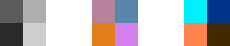As you can see, both img_rgb and img_lab images are of size $2 \times 2$ (instead of $2 \times 2 \times 3$ or $3 \times 2 \times 2$); a RGB image is an array of RGB pixels whereas a Lab image is an array of Lab pixel.

Note

It's recommended to use Gray instead of the Number type in JuliaImages since it indicates that the array of numbers is best interpreted as a grayscale image. For example, it triggers Atom/Juno and Jupyter to display the array as an image instead of a matrix of numbers. There's no performance overhead for using Gray over Number.

This design choice facilitates generic code that can handle both grayscale and color images without needing to introduce extra loops or checks for a color dimension. It also provides more rational support for 3d grayscale images–which might happen to have size 3 along the third dimension–and consequently helps unify the "computer vision" and "biomedical image processing" communities.

## Color conversions are construction/view

Conversions between different Colorants are straightforward:

julia> RGB.(img_gray) # Gray => RGB
2×2 Array{RGB{Float64},2} with eltype RGB{Float64}:
RGB{Float64}(0.355822,0.355822,0.355822)  …  RGB{Float64}(0.682579,0.682579,0.682579)
RGB{Float64}(0.165132,0.165132,0.165132)     RGB{Float64}(0.806625,0.806625,0.806625)

julia> Gray.(img_rgb) # RGB => Gray
2×2 Array{Gray{Float64},2} with eltype Gray{Float64}:
Gray{Float64}(0.583623)  Gray{Float64}(0.484927)
Gray{Float64}(0.566179)  Gray{Float64}(0.648816)
Note

You'll see broadcasting semantics used in JuliaImages here and there, check the documentation if you're not familiar with it.

Sometimes, to work with other packages, you'll need to convert a $m \times n$ RGB image to $m \times n \times 3$ numeric array and vice versa. The functions channelview and colorview are designed for this purpose. For example:

julia> img_CHW = channelview(img_rgb) # 3 * 2 * 2
3×2×2 reinterpret(Float64, ::Array{RGB{Float64},3}):
[:, :, 1] =
0.708011  0.887999
0.515411  0.494824
0.608607  0.0895199

[:, :, 2] =
0.34124   0.833012
0.52376   0.498856
0.661835  0.937867

julia> img_HWC = permutedims(img_CHW, (2, 3, 1)) # 2 * 2 * 3
2×2×3 Array{Float64,3}:
[:, :, 1] =
0.708011  0.34124
0.887999  0.833012

[:, :, 2] =
0.515411  0.52376
0.494824  0.498856

[:, :, 3] =
0.608607   0.661835
0.0895199  0.937867
julia> img_CHW = permutedims(img_HWC, (3, 1, 2)) # 3 * 2 * 2
3×2×2 Array{Float64,3}:
[:, :, 1] =
0.708011  0.887999
0.515411  0.494824
0.608607  0.0895199

[:, :, 2] =
0.34124   0.833012
0.52376   0.498856
0.661835  0.937867

julia> img_rgb = colorview(RGB, img_CHW) # 2 * 2
2×2 reshape(reinterpret(RGB{Float64}, ::Array{Float64,3}), 2, 2) with eltype RGB{Float64}:
RGB{Float64}(0.708011,0.515411,0.608607)   …  RGB{Float64}(0.34124,0.52376,0.661835)
RGB{Float64}(0.887999,0.494824,0.0895199)     RGB{Float64}(0.833012,0.498856,0.937867)
Warning

Don't overuse channelview because it loses the colorant information by converting an image to a raw numeric array.

It's very likely that users from other languages will have the tendency to channelview every image they're going to process. Unfamiliarity of the pixel concept provided by JuliaImages doesn't necessarily mean it's bad.

Note

The reason we use CHW (i.e., channel-height-width) order instead of HWC is that this provides a memory friendly indexing mechanisim for Array. By default, in Julia the first index is also the fastest (i.e., has adjacent storage in memory). For more details, please refer to the performance tip: Access arrays in memory order, along columns

You can use PermutedDimsArray to "reinterpret" the orientation of a chunk of memory without making a copy, or permutedims if you want a copy.

For Gray images, the following codes are almost equivalent except that the construction version copies the data while the view version doesn't.

img_num = rand(4, 4)

img_gray_copy = Gray.(img_num) # construction
img_num_copy = Float64.(img_gray_copy) # construction

img_gray_view = colorview(Gray, img_num) # view
img_num_view = channelview(img_gray_view) # view

## The 0-to-1 intensity scale

In JuliaImages, by default all images are displayed assuming that 0 means "black" and 1 means "white" or "saturated" (the latter applying to channels of an RGB image).

Perhaps surprisingly, this 0-to-1 convention applies even when the intensities are encoded using only 8-bits per color channel. JuliaImages uses a special type, N0f8, that interprets an 8-bit "integer" as if it had been scaled by 1/255, thus encoding values from 0 to 1 in 256 steps.

N0f8 numbers (standing for Normalized, with 0 integer bits and 8 fractional bits) obey standard mathematical rules, and can be added, multiplied, etc. There are types like N0f16 for working with 16-bit images (and even N2f14 for images acquired with a 14-bit camera, etc.).

julia> img_n0f8 = rand(N0f8, 2, 2)
2×2 reinterpret(N0f8, ::Array{UInt8,2}):
0.863  0.882
0.275  0.071

julia> float.(img_n0f8)
2×2 Array{Float32,2}:
0.862745  0.882353
0.27451   0.0705882
Note

This infrastructure allows us to unify "integer" and floating-point images, and avoids the need for special conversion functions (e.g., im2double in MATLAB) that change the value of pixels when your main goal is simply to change the type (numeric precision and properties) used to represent the pixel.

Although it's not recommended, but you can use rawview to get the underlying storage data and convert it to UInt8 (or other types) if you insist.

julia> img_n0f8_raw = rawview(img_n0f8)
2×2 rawview(reinterpret(N0f8, ::Array{UInt8,2})) with eltype UInt8:
0xdc  0xe1
0x46  0x12

julia> float.(img_n0f8_raw)
2×2 Array{Float64,2}:
220.0  225.0
70.0   18.0

Conversions between the storage type, i.e., the actual numeric type, without changing the color type are supported by the following functions:

julia> img = rand(Gray{N0f8}, 2, 2)
2×2 Array{Gray{N0f8},2} with eltype Gray{Normed{UInt8,8}}:
Gray{N0f8}(0.745)  Gray{N0f8}(0.635)
Gray{N0f8}(0.22)   Gray{N0f8}(0.0)

julia> img_float32 = float32.(img) # Gray{N0f8} => Gray{Float32}
2×2 Array{Gray{Float32},2} with eltype Gray{Float32}:
Gray{Float32}(0.745098)  Gray{Float32}(0.635294)
Gray{Float32}(0.219608)  Gray{Float32}(0.0)

julia> img_n0f16 = n0f16.(img_float32) # Gray{Float32} => Gray{N0f16}
2×2 Array{Gray{N0f16},2} with eltype Gray{Normed{UInt16,16}}:
Gray{N0f16}(0.7451)   Gray{N0f16}(0.63529)
Gray{N0f16}(0.21961)  Gray{N0f16}(0.0)

If you don't want to specify the destination type, float is designed for this:

julia> img_n0f8 = rand(Gray{N0f8}, 2, 2)
2×2 Array{Gray{N0f8},2} with eltype Gray{Normed{UInt8,8}}:
Gray{N0f8}(0.804)  Gray{N0f8}(0.643)
Gray{N0f8}(0.345)  Gray{N0f8}(0.298)

julia> img_float = float.(img_n0f8) # Gray{N0f8} => Gray{Float32}
2×2 Array{Gray{Float32},2} with eltype Gray{Float32}:
Gray{Float32}(0.803922)  Gray{Float32}(0.643137)
Gray{Float32}(0.345098)  Gray{Float32}(0.298039)

For a view-like conversion without new memory allocation, of_eltype in MappedArrays is designed for this:

julia> using MappedArrays

julia> img_float_view = of_eltype(Gray{Float32}, img_n0f8)
2×2 mappedarray(x->MappedArrays.convert($(Expr(:static_parameter, 1)), x), y->MappedArrays.convert($(Expr(:static_parameter, 1)), y), ::Array{Gray{N0f8},2}) with eltype Gray{Float32}:
Gray{Float32}(0.803922)  Gray{Float32}(0.643137)
Gray{Float32}(0.345098)  Gray{Float32}(0.298039)

julia> eltype(img_float_view)
Gray{Float32}

## Arrays with arbitrary indices

If you have an input image and perform some kind of spatial transformation on it, how do pixels/voxels in the transformed image match up to pixels in the input? Through Julia's support for arrays with indices that start at values other than 1, it is possible to allow array indices to represent absolute position in space, making it straightforward to keep track of the correspondence between location across multiple images. More information can be found in Keeping track of location with unconventional indices.

## Function categories

See Summary and function reference for more information about each of the topics below. The list below is accessible via ?Images from the Julia REPL. If you've used other frameworks previously, you may also be interested in the Comparison with other image processing frameworks. Also described are the ImageFeatures.jl and ImageSegmentation.jl packages, which support a number of algorithms important for computer vision.

Constructors, conversions, and traits:

• Construction: use constructors of specialized packages, e.g., AxisArray, ImageMeta, etc.
• "Conversion": colorview, channelview, rawview, normedview, PermutedDimsArray, paddedviews
• Traits: pixelspacing, sdims, timeaxis, timedim, spacedirections

Contrast/coloration:

• clamp01, clamp01nan, scaleminmax, colorsigned, scalesigned

Algorithms:

• Reductions: maxfinite, maxabsfinite, minfinite, meanfinite, sad, ssd, integral_image, boxdiff, gaussian_pyramid
• Resizing and spatial transformations: restrict, imresize, warp
• Filtering: imfilter, imfilter!, imfilter_LoG, mapwindow, imROF, padarray
• Filtering kernels: Kernel. or KernelFactors., followed by ando, guassian2d, imaverage, imdog, imlaplacian, prewitt, sobel
• Exposure : build_histogram, adjust_histogram, imadjustintensity, imstretch, imcomplement, AdaptiveEqualization, GammaCorrection, cliphist
• Gradients: backdiffx, backdiffy, forwarddiffx, forwarddiffy, imgradients
• Edge detection: imedge, imgradients, thin_edges, magnitude, phase, magnitudephase, orientation, canny
• Corner detection: imcorner, harris, shi_tomasi, kitchen_rosenfeld, meancovs, gammacovs, fastcorners
• Blob detection: blob_LoG, findlocalmaxima, findlocalminima
• Morphological operations: dilate, erode, closing, opening, tophat, bothat, morphogradient, morpholaplace, feature_transform, distance_transform
• Connected components: label_components, component_boxes, component_lengths, component_indices, component_subscripts, component_centroids
• Interpolation: bilinear_interpolation

• shepp_logan